# Combining Function Transformations: Order Matters

Last time we looked at questions about how to shift, stretch, or flip a graph by changing the equation of a function. All our examples involved only a single transformation. Now we can look at cases where two or more transformations are combined. As we do this, we will develop a deeper understanding of how each transformation works, and how they interact.

## Order of transformations

We’ll spend most of our time with the following question, from 2004:

Order of Transformations of a Function

Could you please tell me in what order I would perform transformations such as -f(x), f(-x), af(x), f(ax), f(x)+a, f(x+a) if two or more were to be applied to f(x)?  As an example, if I had f(ax+b) would I do the
translation or the stretch first?

I've looked in many textbooks and have been unable to find an answer.

Ozgur has learned about each individual transformation (respectively, vertical and horizontal reflections, vertical and horizontal stretches or shrinks, and vertical and horizontal shifts); but now wants to be able to read a function and determine the correct sequence of transformations. Which comes first? (And the example he gives is the hardest case.)

Since this is a favorite topic of mine, I answered:

Good question!  I haven't seen this treated well in textbooks, either, but it's an important topic.

You can perform transformations in any order you want, in general.  But in this case, you are asking in which order to do them in order to transform f(x) into a specific goal, f(ax+b).  The order makes a difference in how you get there.

What I do is to explicitly write the steps, one at a time.  Suppose we first do the horizontal shrink f(x) -> f(ax).  If we then apply a horizontal shift (translation) b units to the left, we would be REPLACING x in f(ax) with x+b, and we'd get f(a(x+b)).  That is NOT what we are looking for; it's equal to f(ax+ab).  So this order of doing those particular transformations is wrong.

I will be reiterating the key idea several times: the horizontal transformations (which affect the input to the function) should be thought of as replacing x with a new expression. I’ll also be emphasizing later some details on what each transformation does to the graph. As I said here, transformations can be applied in any order, but changing the order changes the result, so the trick is to find the order that results in the desired transformed function.

Here, shrinking first, changing $$f(x)$$ to $$g(x) = f(ax)$$, and then shifting,  $$h(x) = g(x + b) = f(a(x+b))$$, didn’t result in the desired function $$h(x) = f(ax+b)$$.

If instead we first do the shift, changing f(x) to f(x+b), and THEN do the shrink, we replace x in x+b with ax, and get f(ax+b), which is what we want.  So that is one answer:

Shift b units to the left:            f(x+b)
Shrink horizontally by a factor of a: f(ax+b)

When I want to be sure of the order, I always take it step by step like this.

But in fact we COULD do the two transformations in the other order, if we change the particular amounts.  We can write f(ax+b) as f(a(x+b/a)), factoring out the a, and then do this:

Shrink horizontally by a factor of a: f(ax)
Shift b/a units to the left:          f(a(x+b/a)) = f(ax+b)

We would do this because of what the first example showed, that this order resulted in the factored form, with parentheses, so we used that form. As we’ll see later, some books teach this form as their routine method; I approve of that because this order of transformations works better for many students.

There are a couple things to notice here.  First, let's visualize what each pair of transformations does.  The first takes the graph of f and moves it left; then shrinks it:

|               |             |
|         /     |       /     |    /
|    +---+      |  +---+      | +-+
|   /           | /           |/
|  /            |/            |/
+----------     +----------   +----------

The other shrinks first, and then shifts--but not as far, since the shrink reduced the distance it has to go:

|               |             |
|         /     |     /       |    /
|    +---+      |  +-+        | +-+
|   /           | /           |/
|  /            | /           |/
+----------     +----------   +----------

To put it another way, the shrink in the second version also moved the starting point of the graph I drew (by shrinking the empty space), so I had to shift it less to get to the destination graph.

Let’s look at actual graphs of a specific function. I’ll start with $$f(x) = x^3$$ (red), and aim for $$h(x) = (\frac {x}{2}-1)^3$$ (blue). The shift-first method is to shift right 1 unit (purple), then stretch by a factor of 2, both numbers being read from the given form: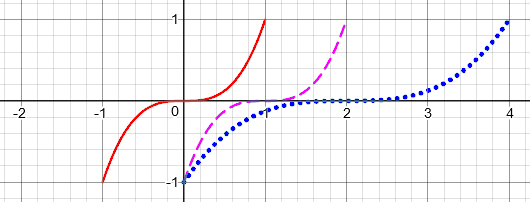The stretch-first method is based on rewriting the function as $$h(x) = (\frac {x-2}{2})^3$$. We first stretch by a factor of 2, then shift right by 2 units: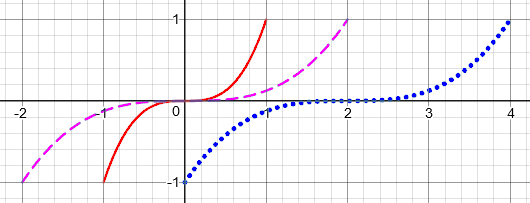Many students find it more natural to do the stretch first, especially when they are reversing the problem, trying to recognize the transformations given the graph (a problem we’ll be looking at next time). When you carry out the stretch in the first form, you must be aware that the stretch starts at the axis, doubling distances from there, rather than from the “center” of the basic graph. As a result, the “center” of the graph ends up moving farther than the shift would suggest (to 2, rather than just to 1).

A second point to make is that the order of operations determines the order of the transformations.  When we wrote the transformed function as f(ax+b), the operations inside the function were done in the order "multiply, then add"; since we are replacing x in each transformation, we ended up doing the last operation first (outside in), replacing x with x+b first, then with ax, so we did the shift followed by the shrink.  When we wrote it as f(a(x+b/a)), the order of operations says the addition comes first, then the multiplication, so the transformations have to be done in the opposite order.

This applies to transformations of x, on the inside of the function. Transformations like a+bf(x) (vertical shifts and stretches) are done in the SAME order as the order of operations.  Also, these do not interact with the horizontal transformations, so it doesn't matter which order you do them in; if you had, say, af(bx) you could do the vertical stretch followed by the horizontal shrink, or vice versa.  Try it and see!

We can summarize the order of transformations this way: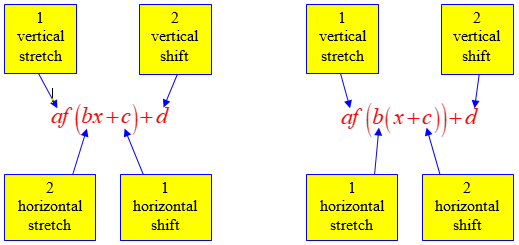I haven’t really discussed reflections yet, but since they also amount to multiplication (by -1), they are done along with the stretch.

## A specific example

In 2007, Elizabeth wrote asking for clarification:

I read your explanation of transformations order of operations, but I guess I'm still unclear on the order that I have to do them in.  For example, if I take the equation y = 4 sqrt(2-x), I find that I get the correct graph by doing
1) reflection over y axis
2) horizontal shift of 2
3) vertical stretch of 4
OR
1) vertical stretch
2) reflection
3) horizontal shift.
Either way, the horizontal shift has to come after the reflection.

It doesn't work if I
1) shift then
2) reflect or stretch
3) reflect or stretch (depending on what I did in step 2).
I was always taught to do the horizontal shift first!

According to your explanation, as long as what I'm doing yields the correct final equation, I can do whatever order I need to get there. So looking at what didn't work, if I shift first I get sqrt(x-2). Then reflect makes sqrt(-1)(x-2) or in other words sqrt(-x+2) which is the same as sqrt(2-x), then finally vertical stretch yields what I want:  y = 4 sqrt(2-x).  But this doesn't graph correctly; the reflection must come before I do the horizontal shift. Why?

Her example is a little more complicated because of the additional reflection; it will be a welcome addition here, to make everything more concrete. I replied:

Your question brings up details I didn't emphasize in my original answer.

First, I want to point out clearly what each individual transformation looks like, because there are a few points that are easily missed. Here are the transformations mentioned on that page:

-f(x)     reflection in the x-axis
af(x)     vertical stretch by factor a
f(x)+a    vertical shift up by a

f(-x)     reflection in the y-axis
f(ax)     horizontal shrink by factor a
f(x+a)    horizontal shift left by a

Note that the first set, the "vertical" transformations, involve changing something OUTSIDE the original function; that is, we do something to the "y" that comes out. The second set, the "horizontal" transformations, involve changing something on the INSIDE of the function. This MUST be seen as REPLACING the x itself with a negative, multiple, or sum. These are the tricky ones. (They are also the ones that tend to act in the opposite direction to what many people expect, shrinking or shifting left rather than stretching or shifting right.)

Last time, I pointed out the idea of replacing x, but it only becomes essential here, with a combination of horizontal transformations. Her example is a perfect one to demonstrate:

Let's take an example of a reflection in the y-axis for a relatively complicated (compound) function. If

f(x) = sqrt(x - 2)

then reflection in the y-axis involves NOT replacing x-2 with its opposite, 2-x, but replacing x with its opposite:

g(x) = f(-x) = sqrt(-x-2)

Try graphing this to see for yourself what happens. A point (x,y) that satisfies y=f(x), reflected in the y-axis to the point (-x,y), will satisfy y=g(x) (using the new value of x). If you graph sqrt(2 - x), you'll see something different.

This, again, is the key point: $$f(-x)$$ doesn’t mean “multiply the argument of the square root by -1”; it means “replace x with –x“.

Again, making one transformation at a time makes this clearer:

Now, let's break your function down into a series of transformations, starting with the basic square root function:

f1(x) = sqrt(x)

f(x) = 4 sqrt(2 - x)

It doesn't matter how the vertical and horizontal transformations are ordered relative to one another, since each group doesn't interact with the other. Let's do all the horizontal transformations first, since they're the awkward ones. We need to do something with the 2, and something with the -x. As mentioned in the page above, it's most convenient (because of the order of operations) to do the shift FIRST:

f1(x) = sqrt(x)
f2(x) = f1(x + 2) = sqrt(x + 2)   shift left by 2
f3(x) = f2(-x) = sqrt(-x + 2)     reflect in y-axis

Now we have f3(x) = sqrt(2 - x), and we can apply the vertical transformation:

f4(x) = 4 f3(x) = 4 sqrt(2 - x)   stretch vertically by 4

As before, I’m doing the shift first primarily because of the way the function is written. I could instead have first rewritten it with the function argument in factored form, as I will do later.

In terms of the order of operations, our function looks like this: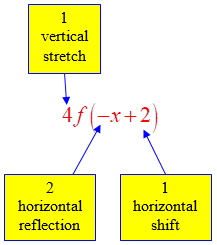So we find that we can shift left by 2, then reflect in the y-axis, and then stretch vertically by 4. And that sequence of transformations works on the graph:

*       |
|
*    |
|        *          |       *        *       |                 |
|   *        -->    |  *        -->       *  |    -->       *  |
|*                 *|                        |*                |
*---------        *-+--------        --------+-*              *|
|
|*
|
| *
|
--------+-*

This is the right graph; for example, f(2) = 4 sqrt(2 - 2) = 0, and f(0) = 4 sqrt(2 - 0) = 4 sqrt(2) = 5.6.

Here is a better graph, showing the transformation from $$f_1(x)=\sqrt{x}$$ (red) to $$f_2(x)=\sqrt{x+2}$$ (purple) to $$f_3(x)=\sqrt{-x+2}$$ (dotted blue) to $$f_4(x)=4\sqrt{2-x}$$ (solid blue):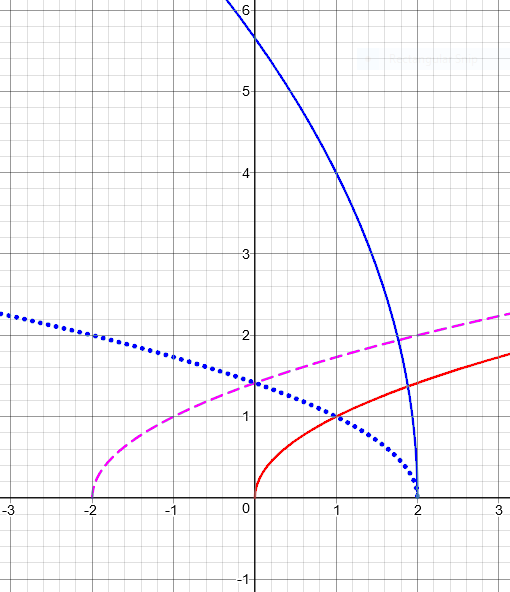Note how I checked my graph by choosing a point; after drawing the graph, I might observe that (1, 4) is on the final graph, and verify that $$f_4(1) = 4\sqrt{2-1} = 4$$ as it should.

I closed by commenting on Elizabeth’s description in her last paragraph:

You seem to have fallen into the trap I mentioned, negating the entire argument of the sqrt, rather than replacing x with -x, and thinking that you have reflected the graph in the y-axis. Be sure to graph the function you got, and convince yourself that it is not the reflection you think it is. The functions sqrt(x-2) and sqrt(2-x) both have x-intercept x=2, so in fact you have reflected around the line x=2!

Similarly, I suspect that when you did the shift after the reflection, you were doing some wrong transformations, and not actually graphing what you got and seeing that it really worked. Please let me know if I'm wrong about that.

She responded,

Aha!  You are absolutely correct.  [of course :) ]  I was improperly applying the transformation.  Your key phrase "This MUST be seen as REPLACING the x itself with a negative, multiple or sum" cleared it up for me.  Thank you for your time and clear explanation!

This 2007 page gives another example:

Does the Order Matter When Transforming a Function?

## Looking at the graph

The next question, from 2017, faces the issue I mentioned about seeing the transformations of the graph incorrectly.

Order of Transformations of a Function, Redux

I'm having difficulty interpreting combinations of horizontal shifts, shrinks, and stretches. I understand how they work individually, such as how the scalar in 3x^2 makes the function three times steeper, etc. But how would you solve a combination of them together?

I saw a related Dr. Math conversation transform shifts in this order:

Shift b units to the left: f(x + b).
Shrink horizontally by a factor of a: f(ax + b).

Let's say we have the equation f(x) = (3x - 9)^2.

I think the steps of the transformations would be:

Replace x by (x - b): f(x - b). In this case, it would be x - 9.    Finally, replace the x in f(x - b) with ax. In this case, it would be    3, which would result in

f(3x - 9) = (3x - 9)^2

My reasoning is that it would shift 9 spaces and THEN shrink 3 times its original size. BUT the graph of f(x) = (3x - 9)^2 shows that it only shifted 3 units to the left, not 9.

I am confused why it shifts only 3 spaces. Why not nine? I don't understand how this works!

All of the work here is correct, except for the misunderstanding of what the graph shows. I replied:

You correctly broke down the transformations of the function; but you are not looking at the transformed graph properly. This is a common difficulty (especially in drawing the graph by hand, or finding the shift in a given graph), and for this reason I prefer to change the order -- it makes the graph easier to work with. So I'll first look at what you are doing, and then do it differently.

You know that the graph is shifted 9 units to the right; but this is BEFORE the shrink. When you shrink the resulting graph, you are expecting the graph to still show the same shift.

But in fact, the shift itself has been shrunk. What is happening is that every point, including the vertex that you are looking at, is being moved to 1/3 as far from the y-axis as it was; so what WAS a shift by 9 units is now only 3 units.

Ayush is looking at the graph and seeing a shift of only 3 units; but that is the shift you would have done if you shrunk the graph first and then shifted.

Here is the graph of what Ayush has done, showing the intermediate step of shifting right by 9 units (purple) before shrinking (blue):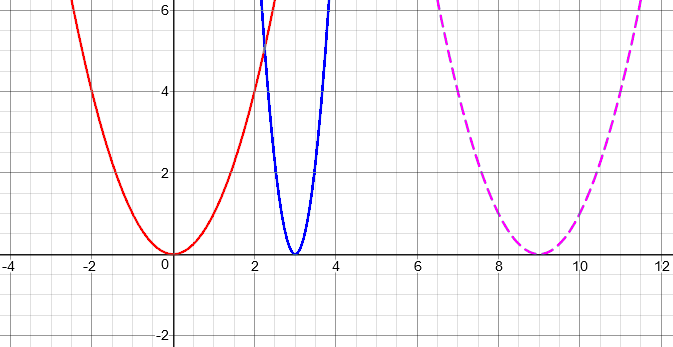To see this, consider how each transformation moves the point (0, 0) in the original graph:

Shift 9 units to the right: (9, 0)
Shrink horizontally by a factor of 3: (3, 0)

So the vertex is only 3 units to the right of where it was, because the shift of 9 was divided by 3.

Of course, just checking a couple points can also confirm that the graph is correct, even if you are unsure of the transformations. But there’s more:

What you are doing, which I find most students naturally do, is to picture the shrinking as centered on the axis of the graph, as a real object would. But the transformation shrinks the distance of every point from the y-axis -- not the distance from the axis of the parabola. When you shrink an already-shifted graph, your mind does not want to see it that way! So, as I mentioned, I like to arrange things so that we do the shrink first, which is easier to see.

So consider what happens if we rewrite the function. I'll call it g to distinguish it from the original function f(x) = x^2.

Factoring out the 3 on the inside,

g(x) = (3x - 9)^2
= f(3x - 9)
= f(3(x - 3))
= (3(x - 3))^2

Do you see how the 9 became a 3? By changing the order of operations using the parentheses, we have also changed the order of the transformations:

Shrink horizontally by 3: f(3x)         (3x)^2         (0, 0)
Shift 3 units to the right: f(3(x - 3))   (3(x - 3))^2   (3, 0)

Here we first replaced x with 3x, which shrinks the graph about the y-axis; then we replaced x with (x - 3), which shifts the graph right. Since the point of reference, the vertex, was still on the y-axis when we did the shrink, it was not affected; and now the effect of the shift is directly visible.

Here is a graph of this process, where we first shrink (purple) and then shift (blue):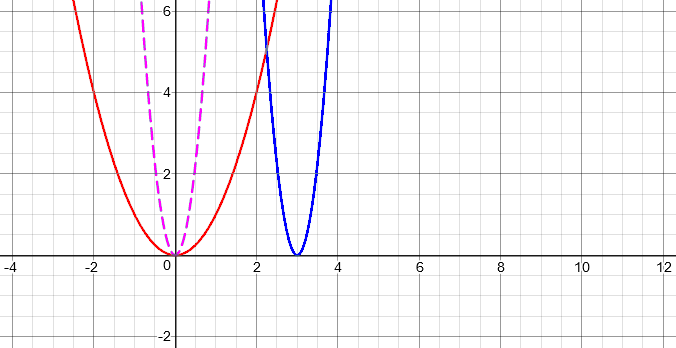It is this shift that people tend to see when they look at a graph, because we think of the graph as getting thinner with respect to itself, not of the whole plane shrinking toward the axis.

### 11 thoughts on “Combining Function Transformations: Order Matters”

1.Love the yellow diagrams. So helpful for bringing all the concepts together.

2.Excellent post! My signals professor said “order doesn’t matter, but sometimes it does” when graphing. Thank you for giving a coherent answer!

3.This is exactly what I was looking for! I struggled figuring out why the order of f(ax+b) and f(a(x+b/a)) were different. Order of operations backwards, just like solving a two step equation.

4.thank you so so so so so much 🙏 answered every single question I had

5.Brilliant article. What level of maths were these students? Was this aimed at university students?

1.I have no records to see how old these particular students were; but the material is high-school level (pre-calculus), and I’ve taught it in community college (as preparation for college-level classes). My approach to it, however, is perhaps deeper than many textbooks at this level, because I think more is needed in order to fully understand than is commonly taught. (Some of the other posts on this topic explicitly explore underlying ideas like function composition.)

This site uses Akismet to reduce spam. Learn how your comment data is processed.